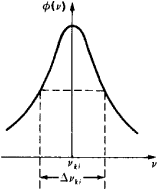# Spectral Lines, Width of

## Spectral Lines, Width of

Spectral lines in optical spectra of atoms, molecules, and other quantum systems are characterized by a range of frequencies v or a range of wavelengths λ = c/v, where c is the speed of light. Such a frequency or wavelength range is called the width of spectral lines.

A certain range Δvki of frequencies near the frequency vki of a radiative transition between discrete energy levels ℰk and ℰi corresponds to every such transition; the transition frequency vMki = (ℰk - ℰi)lh = (ℰk - ℰj)/2πhℏ, where h = 2πℏ is Planck’s constant. The value of Δvki gives the width of a spectral line, that is, the extent to which a given spectral line is nonmonochromatic. A spectral-line profile φ(v)—that is, the frequency dependence of the intensity of emission or absorption—usually has a maximum at or near the transition frequency vki (see Figure 1). The frequency range between points where the intensity falls to half the maximum intensity is taken as the width of a spectral line. Hence, the width of a spectral line is often referred to as the half-width of a spectral line. If the Doppler effect is not taken into account, the width Δvki of a spectral line is given by the sum of the widths of the energy levels ℰk and ℰi: Δvki = (Δℰk – Δℰi)\h ≈ (1/τk + 1/τi)/2π. In other words, the shorter the lifetimes τk and τi at the energy levels, the greater the value of Δvki.Figure 1. A symmetrical spectral-line profile. The maximum emission intensity φ(v) corresponds to the frequency vki; the width of the spectral line (Δvki) is equal to the frequency range between the points where the intensity falls to half the maximum intensity.

The natural width of a spectral line is given by the expression (Δvki)nat = (Ak + Ai)/2π, where Ak and Ai are the total probabilities of spontaneous transitions from the levels ℰk and ℰi to all lower-lying levels. The natural width is very small.

For atoms and molecules, the width of spectral lines is governed mainly by the broadening of the energy levels of the atoms or molecules during interactions with surrounding particles and by the broadening of the spectral lines as a result of the Doppler effect. In a gas or a plasma, the energy levels of the particles are broadened during collisions. Depending on the type of broadening, a symmetrical or an asymmetrical spectral-line profile is produced. Figure 1 shows a symmetrical profile, which is characteristic of radiation broadening.

### REFERENCES

Heitler, W. Kvantovaia teoriia izlucheniia. Moscow, 1956. (Translated from English.)
Sobel’man, I. I. Vvedenie v teoriiu atomnykh spektrov, 2nd ed. Moscow, 1977.

M. A. EL’ASHEVICH

Site: Follow: Share:
Open / Close